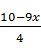### Sample Problem

Which of the following correctly solves the linear equation 9x + 4y – 3 = 7 for y?

#### Solution

9x + 4y – 3 = 7

4y + 9x = 10

4y = 10 – 9x

y =